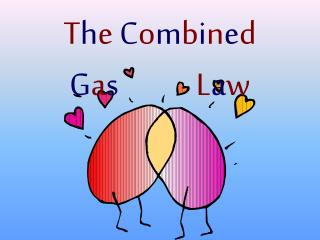DownloadDownload PresentationT h e C o m b i n e d G a s L a w

# T h e C o m b i n e d G a s L a w

Télécharger la présentation## T h e C o m b i n e d G a s L a w

- - - - - - - - - - - - - - - - - - - - - - - - - - - E N D - - - - - - - - - - - - - - - - - - - - - - - - - - -
##### Presentation Transcript

1. The CombinedGas Law

2. Manipulating Variables in equations • Often in an equation we want to isolate some variable, usually the unknown • From math: what ever you do to one side of an equation you have to do to the other side • Doing this keeps both sides the same • E.g. x + 5 = 7, what does x equal? • We subtract 5 from both sides … • x + 5 – 5 = 7 – 5, thus x = 2 • Alternatively, we can represent this as 5 moving to the other side of the equals sign … • x + 5 = 7 becomes x = 7 – 5 or x = 2 • Thus, for addition or subtraction, when you change sides you change signs

3. Multiplication and division • We can do a similar operation with multiplication and division • E.g. 5x = 7, what does x equal? • We divide each side by 5 (to isolate x) … • 5x/5 = 7/5 … x = 7/5 … x = 1.4 • Alternatively, we can represent this as 5 moving to the other side of the equals sign … • 5x = 7 becomes x = 7/5 • Thus, for multiplication and division, when you change sides you change position (top to bottom, bottom to top)

4. (x) (y) 7a = 5 b (x) (y) 7a = 5 b (x)(y)(b) 7a = 5 (x)(y)(b) (x)(y)(b) = a a = (35) (5)(7) Multiplication and division • Let’s look at a more complicated example: • Isolate a in the equation: • Move b to the other side (from bottom to top) • Move 7 to the other side (from top to bottom) or

5. (x) (y) 7a = 5 b (x) (y) 7a = 5 b 35a (b)(x)(y) 7a b = = xy 5 P1V1 P2V2 T1 T2 = Multiplication and division • This time, isolate b in the equation: • Move b to the other side (it must be on top) … • Move everything to the other side of b Q - Rearrange the following equation to isolate each variable (you should have 6 equations)

6. P1T2V1 P1T2V1 P2T1V2 P1T2V1 P2T1V2 P2T1V2 P2 T1 T2 V1 P1 V2 = = = = = = P2V2 T1V2 P1V1 P2T1 T2P1 T2V1 Combined Gas Law Equations

7. V1 P1 V2 P2 P1V1 = P2V2 = = T1 T1 T2 T2 P1V1 P2V2 T1 T2 = Combining the gas laws • So far we have seen two gas laws: Robert Boyle Jacques Charles Joseph Louis Gay-Lussac These are all subsets of a more encompassing law: the combined gas law Read pages 437, 438. Do Q 26 – 33 (skip 31)

8. P1V1 P2V2 = T1 T2 (101 kPa)(50.0 mL) (P2)(12.5 mL) = (T1) (T2) (101 kPa)(50.0 mL)(T2) = 404 kPa (P2) = (T1)(12.5 mL) Q 26 V1 = 50.0 ml, P1 = 101 kPa V2 = 12.5 mL, P2 = ? T1 = T2 Notice that T cancels out if T1 = T2

9. P1V1 P2V2 = T1 T2 (P1)(0.10 L) (P2)(V2) = (298 K) (463) (P1)(0.10 L)(463 K) = 0.16 L (V2) = (P2)(298 K) Q 27 V1 = 0.10 L, T1 = 298 K V2 = ?, T2 = 463 P1 = P2 Notice that P cancels out if P1 = P2

10. P1V1 P2V2 = T1 T2 (150 kPa)(V1) (250 kPa)(V2) = (308 K) (T2) (250 kPa)(V2)(308 K) = 513 K = 240 °C (T2) = (150 kPa)(V1) Q 28 P1 = 150 kPa, T1 = 308 K P2 = 250 kPa, T2 = ? V1 = V2 Notice that V cancels out if V1 = V2

11. P1V1 P2V2 = T1 T2 (100 kPa)(5.00 L) (90 kPa)(V2) = (293 K) (308 K) (100 kPa)(5.00 L)(308 K) = 5.84 L (V2) = (90 kPa)(293 K) Q 29 P1 = 100 kPa, V1 = 5.00 L, T1 = 293 K P2 = 90 kPa, V2 = ?, T2 = 308 K Note: although kPa is used here, any unit for pressure will work, provided the same units are used throughout. The only unit that MUST be used is K for temperature.

12. P1V1 P2V2 = T1 T2 (800 kPa)(1.0 L) (100 kPa)(V2) = (303 K) (298 K) (800 kPa)(1.0 L)(298 K) = 7.9 L (V2) = (100 kPa)(303 K) Q 30 P1 = 800 kPa, V1 = 1.0 L, T1 = 303 K P2 = 100 kPa, V2 = ?, T2 = 298 K

13. P1V1 P2V2 = T1 T2 (6.5 atm)(2.0 mL) (0.95 atm)(V2) = (283 K) (297 K) (6.5 atm)(2.0 mL)(297 K) = 14 mL (V2) = (0.95 atm)(283 K) Q 32 P1 = 6.5 atm, V1 = 2.0 mL, T1 = 283 K P2 = 0.95 atm, V2 = ?, T2 = 297 K 33. The amount of gas (i.e. number of moles of gas) does not change. For more lessons, visit www.chalkbored.com